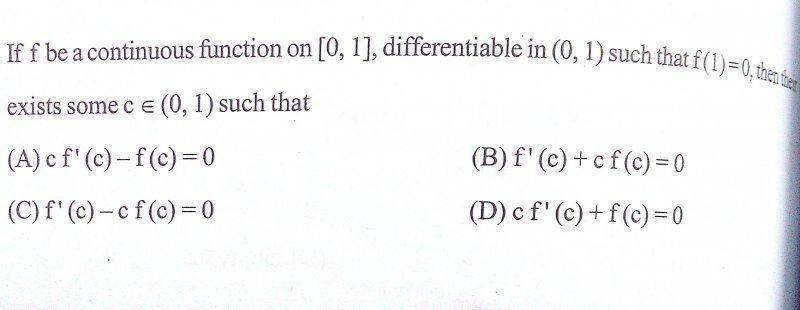# Lagrange's mean value theorem problem

## Homework Statement## Homework Equations

Lagrange's mean value theorem

## The Attempt at a Solution

Applying LMVT,
There exists c belonging to (0,1) which satisfies f'(c) = f(1)-f(0)/1 = -f(0)
But this gets me nowhere close to the options... :(

edit
Sorry the post I made contained an error. I'm not quite sure how to fix it yet but basically what I think you need to do is find a function h(x) and define it in terms of some linear combination of f(x), f(0), f(1) so that it equals zero at the end points and then apply Rolle's Theorem.

Thanks mate!! Solved it... But how did you think of that function h(x)??

edit
Sorry the post I made contained an error. I'm not quite sure how to fix it yet but basically what I think you need to do is find a function h(x) and define it in terms of some linear combination of f(x), f(0), f(1) so that it equals zero at the end points and then apply Rolle's Theorem.

Hey sorry I edited my previous post. What I wrote doesn't work because the two c's might be different. The c that works for the equation you posted might be a different c from the one I used for h(x).

When trying to define an h(x) we want it to be 0 at the end points so we can apply Rolle's Theorem. It looks like Rolle's Theorem is needed because of the way the question is set up (i.e it looks like some derivative is equal to 0). And we want f(0) to disappear from the equation for the derivative because we don't know its value.

•utkarsh009
Actually a relatively simple function works lol. Sorry for all this confusion:
$$h(x) = xf(x)$$
There exists a c in (0, 1) such that $$0 = cf'(c) + f(c)$$

by Rolle's Theorem.

•utkarsh009
Actually a relatively simple function works lol. Sorry for all this confusion:
$$h(x) = xf(x)$$
There exists a c in (0, 1) such that $$0 = cf'(c) + f(c)$$

by Rolle's Theorem.
Yah... Was just thinking the same and was about to post it... :P